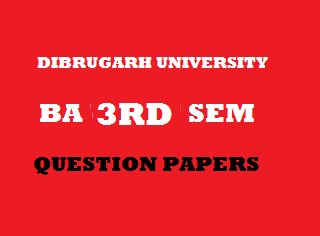## Thursday, January 03, 20192015
(November)
ECONOMICS
(Major)
Course: 301
(Microeconomics - II)
Full Marks: 80
Pass Marks: 32 (Backlog)/24 (2014 onwards)
Time: 3 hours
The figures in the margin indicate full marks for the questions

1. Which of the following is not a characteristic of a ‘price taker’?
1. TR = P x Q.
2. AR = Price.
3. Negatively sloped demand curve.
4. Marginal Revenue = Price.
1. Oligopolistic industries are characterized by
1. A few dominant firms and substantial barriers to entry.
2. A few large firms and no entry barriers.
3. A large number of small firms and no entry barriers.
4. One domain firm and less entry barriers.
1. The long-run equilibrium outcomes in monopolistic competition and perfect competition are similar because in both market structures
1. The efficient output level will be produced in the long run.
2. Firms will be producing at minimum average cost.
3. Firms realize all economies of scale.
4. None of the above.
1. Discriminating monopoly implies that the monopolist charges different prices for his commodity
1. From different groups of consumers.
2. For different uses.
3. At different places.
4. Any of the above.
1. A firm encounters its ‘shutdown point’, when
1. AC = price at the profit maximizing level of output.
2. AVC = price at the profit maximizing level of output.
3. AFC = price at the profit maximizing level of output.
4. MC = price at the loss minimizing level of output.
1. Define general equilibrium.
2. The marginal productivity theory of distribution is
1. An explanation of factor-pricing.
2. An explanation of national income determination.
3. An explanation for personal income distribution.
4. None of the above.

1. In the Ricardian theory of economic rent, transfer earnings of land are presumed to be
1. Equal to rent.
2. Negative.
3. Positive.
4. Zero.
2. Write short notes on any four of the following (within 150 words each): 4x4=16
1. Rules for profit maximization.
2. Price discrimination.
3. Unique characteristics of oligopoly market.
4. Classical theory of interest.
5. Interdependence of markets.
Answer the following questions (within 500 words each):
3. (a) Explain with the help of suitable diagrams, the long-run equilibrium of the firm and the industry under perfect competition. 6+6=12
Or
(b) Describe the process of derivation of the supply curve of the firm and the industry in the short-run under perfect competition. 6+6=12
4. (a) What is Monopsony? Explain the sources of Monopsony power. What are the differences between Monopsony and Monopoly? 2+6+3=11
Or
(b) Explain with examples, the concepts ‘natural monopoly’ and ‘price regulation’ of monopoly.       5+6=11
5. (a) Distinguish monopolistic competition from perfect competition. Prove that firms under monopolistic competition will be less than optimum size in the long run. 4+7=11
Or
(b) What are the differences between monopoly market and oligopoly market? Explain with examples, the types of oligopoly. 4+7=11
6. (a) Discuss the Euler’s theorem of distribution of factor income. 11
Or
(b) Distinguish between rent and quasi rent. Explain the Ricardian theory of rent. 3+8=11
7. (a) Discuss with a diagram, how the competitive markets attain efficiency in exchange. 11
Or
(b) Write explanatory notes on the following: 5+6=11
1. Market failure.
2. Efficiency in production in competitive markets.

***

#### 1 comment:

1.Economic 3rd semester major Questions are not clear so please sir completely give

Kindly give your valuable feedback to improve this website.